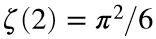# Problem 45939. Estimate π from certain values of the zeta function

Cody Problems 2908 and 2909 ask us to estimate π by summing a given number of terms in an infinite sum, the Leibniz formula.
Here you are asked to estimate π by summing a given number of terms in other infinite sums corresponding to the Riemann zeta function evaluated at positive even integers. For example, in solving the Basel problem, Euler summed the reciprocals of the squares of positive integers and showed that.
Write a function that takes a vector n with the numbers of terms to sum and a vector m with the values at which to estimate the zeta function. The function should return an array of character strings with the absolute value of the relative error of the estimate E--that is, abs(E-pi)/pi. Report the relative error in scientific notation using num2str(...,'%10.2e').
For example, PiByZeta(30,2) should return
'1.00e-02'
while PiByZeta([25 30],[2 8]) should return
'1.20e-02 1.00e-02'
'2.53e-12 7.22e-13'

### Solution Stats

42.86% Correct | 57.14% Incorrect
Last Solution submitted on Sep 11, 2023

### Community Treasure Hunt

Find the treasures in MATLAB Central and discover how the community can help you!

Start Hunting!